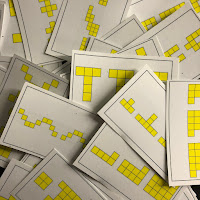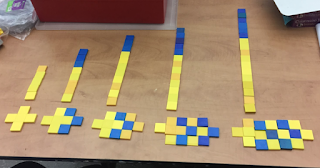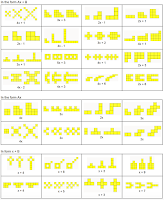## Tuesday, 19 December 2017

### Visual Pattern CardsThis activity was inspired by @JoBoalar's TED talks were she talks about people using colours to show how patterns grow. It was also inspired by @FawnpNguyen's awesome VisualPatterns.org site. The premiss is simple, students are each given a card with three terms of a linear pattern on it. They are to recreate the pattern (and add two more terms) using colour to show how it grows. Then they use what they see to help come up with a general term for their pattern.

For this activity there are 32 cards made that have linear patterns in the form Ax, x + B and Ax + B. For grade 7, if your focus is developing the general term, then you should just use the cards with general terms in the form Ax & x + B (patterns that have expressions involving only one operation).• Gr7PA – represent linear growing patterns, using a variety of tools (e.g., concrete materials, paper and pencil, calculators, spreadsheets) and strategies (e.g., make a table of values using the term number and the term; plot the coordinates on a graph; write a pattern rule using words);
• Gr7PA – make predictions about linear growing patterns, through investigation with concrete materials;
• Gr7PA – develop and represent the general term of a linear growing pattern, using algebraic expressions involving one operation (e.g., the general term for the sequence 4, 5, 6, 7, … can be written algebraically as n + 3, where n represents the term number; the general term for the sequence 5, 10, 15, 20, … can be written algebraically as 5n, where n represents the term number);
• Gr8PA – represent, through investigation with concrete materials, the general term of a linear pattern, using one or more algebraic expressions (e.g.,“Using toothpicks, I noticed that 1 square needs 4 toothpicks, 2 connected squares need 7 toothpicks, and 3 connected squares need 10 toothpicks. I think that for n connected squares I will need 4 + 3(n – 1) toothpicks, because the number of toothpicks keeps going up by 3 and I started with 4 toothpicks. Or, if I think of starting with 1 toothpick and adding 3 toothpicks at a time, the pattern can be represented as 1 + 3n.”);
• Gr8PA – represent linear patterns graphically (i.e., make a table of values that shows the term number and the term, and plot the coordinates on a graph), using a variety of tools (e.g., graph paper, calculators, dynamic statistical software);
• Gr8PA – determine a term, given its term number, in a linear pattern that is represented by a graph or an algebraic equation1. For this activity there are 32 possible cards to use. Print them out on card stock (and laminate them if possible). Cut out each card to create a class set. If you are doing this with grade 7s then you may want two sets of the last two pages of cards since they deal with general terms in the form Ax & x + B.
2. Print out the Answer card so that you can circulate easily giving help or advice.
3. Students should have enough connecting cubes or colour tiles of various colours to create the patterns. Or students could use this virtual colour tiles app from Mathies.ca1. Hand out one card per student (you could also do one card per pair and have extra cards for students that finish early)
2. Instruct students to recreate their pattern using colours (connecting cubes or colour tiles) to show how the pattern grows. You may also ask them to restrict to two colours and show it that way as well.
3. Once students have recreated their pattern, have them create the next two terms using the same colour distinctions.
4. Once you are satisfied with their five terms, have them re arrange their tiles so that they create a line of each set of tiles for each term (like a bar graph).  While groups are getting to the same place you might ask quicker groups to determine the number of tiles needed for the 15th term
5. Next have students use their tiles to help determine the general term
6. You might want to take several different examples to consolidate creating the general term.
As an alternate procedure you might consider the progression of steps seen in this video using some of these cards to more systematically develop the a method for generating the algebraic expression for a linear pattern.

Did you use this activity? Do you have a way to make it better? If so tell us in the comment section. Thanks